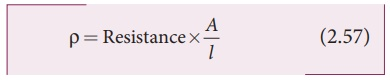Home | | Physics 12th Std | Meter bridge

# Meter bridge

The meter bridge is another form of WheatstoneŌĆÖs bridge.

Meter bridge

The meter bridge is another form of WheatstoneŌĆÖs bridge. It consists of a uniform manganin wire AB of one meter length. This wire is stretched along a meter scale on a wooden board between two copper strips C and D. Between these two copper strips another copper strip E is mounted to enclose two gaps G1 and G2 as shown in Figure 2.26. An unknown resistance P is connected in G1 and a standard resistance Q is connected in G2. A jockey (conducting wire) is connected to the terminal E on the central copper strip through a galvanometer (G) and a high resistance (HR). The exact position of jockey on the wire can be read on the scale. A Lechlanche cell and a key (K) are connected across the ends of the bridge wire.The position of the jockey on the wire is adjusted so that the galvanometer shows zero deflection. Let the point be J. The lengths AJ and JB of the bridge wire now replace the resistance R and S of the WheatstoneŌĆÖs bridge. Thenwhere RŌĆ▓ is the resistance per unit length of wireThe bridge wire is soldered at the ends of the copper strips. Due to imperfect contact, some resistance might be introduced at the contact. These are called end resistances. This error can be eliminated, if another set of readings are taken with P and Q interchanged and the average value of P is found.

To find the specific resistance of the material of the wire in the coil P, the radius r and length l of the wire is measured. The specific resistance or resistivity Žü can be calculated using the relation

Resistance = Žü . l/ABy rearranging the above equation, we getIf P is the unknown resistance equation (2.57) becomes,EXAMPLE 2.25

In a meter bridge with a standard resistance of 15 ╬® in the right gap, the ratio of balancing length is 3:2. Find the value of the other resistance.

SolutionEXAMPLE 2.25

In a meter bridge, the value of resistance in the resistance box is 10 ╬®. The balancing length is l1 = 55 cm. Find the value of unknown resistance.

Solution

Q = 10 Ōä”Tags : Explanation, Formulas, Solved Example Problems | KirchhoffŌĆÖs rule and WheatstoneŌĆÖs bridge , 12th Physics : Current Electricity
Study Material, Lecturing Notes, Assignment, Reference, Wiki description explanation, brief detail
12th Physics : Current Electricity : Meter bridge | Explanation, Formulas, Solved Example Problems | KirchhoffŌĆÖs rule and WheatstoneŌĆÖs bridge